# Lagrange stability

Jump to: navigation, search

A property of a point(a trajectory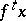) of a dynamical system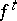(or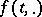, cf. ) given on a metric space, requiring that the trajectoryis contained in a pre-compact set (cf. Pre-compact space).

If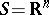, then Lagrange stability is the same as boundedness of the trajectory. If for all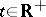(respectively, for all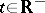) the pointis contained in a pre-compact set, then the trajectory(the point) is called positively (respectively, negatively) Lagrange stable. The concept of Lagrange stability was introduced by H. Poincaré in connection with analyzing the results of J.L. Lagrange on the stability of planetary orbits.

Birkhoff's theorem: Ifis complete, then the closure of a positively or negatively Lagrange-stable trajectory contains at least one compact minimal set. Every point of a compact minimal set is a recurrent point.